Скачать презентацию Evaluation next steps Lift and Costs

97738b4fa36a206b08cdd582b91a8baf.ppt

• Количество слайдов: 32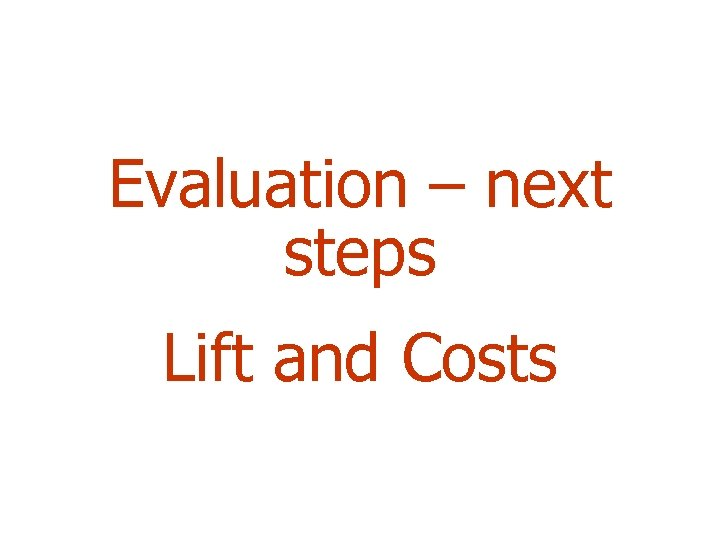Evaluation – next steps Lift and Costs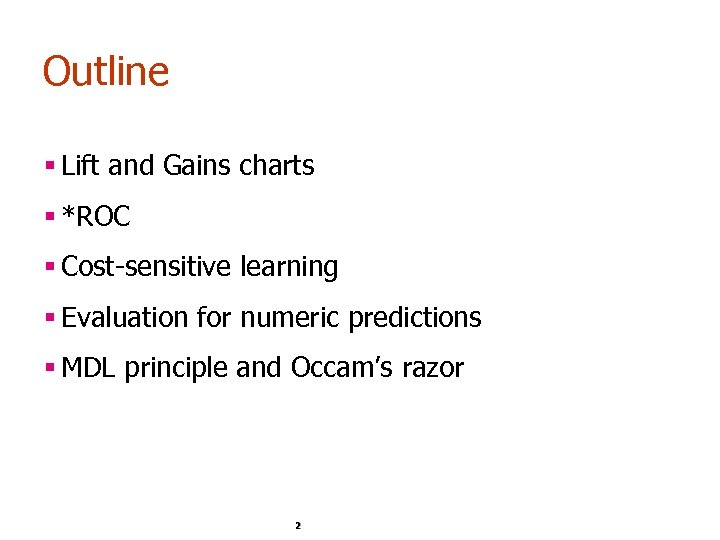Outline § Lift and Gains charts § *ROC § Cost-sensitive learning § Evaluation for numeric predictions § MDL principle and Occam’s razor 2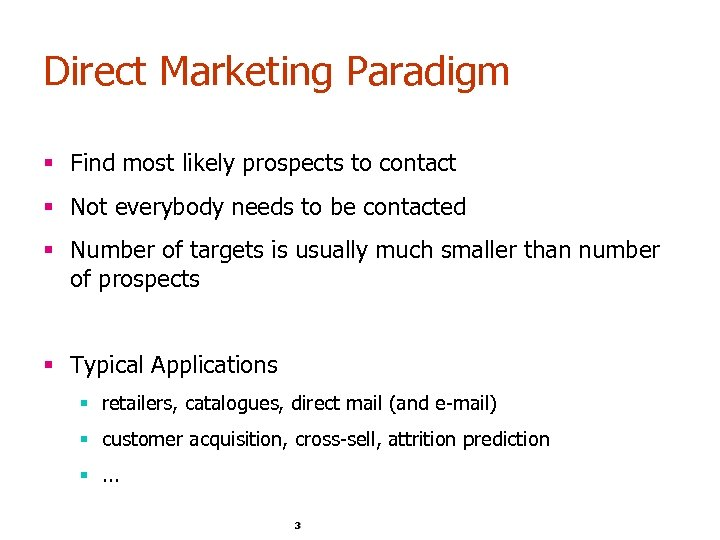Direct Marketing Paradigm § Find most likely prospects to contact § Not everybody needs to be contacted § Number of targets is usually much smaller than number of prospects § Typical Applications § retailers, catalogues, direct mail (and e-mail) § customer acquisition, cross-sell, attrition prediction §. . . 3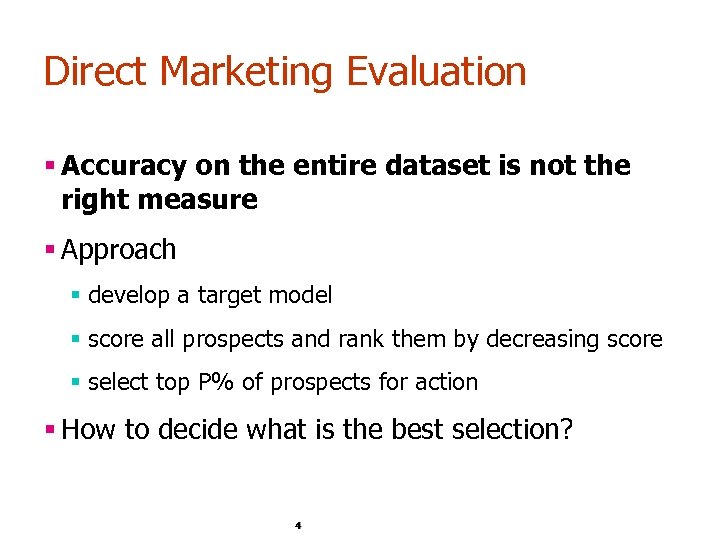Direct Marketing Evaluation § Accuracy on the entire dataset is not the right measure § Approach § develop a target model § score all prospects and rank them by decreasing score § select top P% of prospects for action § How to decide what is the best selection? 4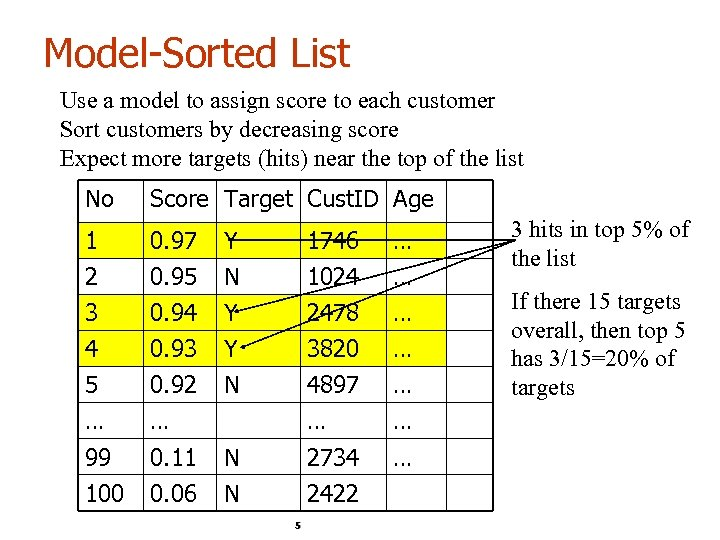Model-Sorted List Use a model to assign score to each customer Sort customers by decreasing score Expect more targets (hits) near the top of the list No Score Target Cust. ID Age 1 2 3 0. 97 0. 95 0. 94 Y N Y 1746 1024 2478 … … … 4 5 … 0. 93 0. 92 … Y N 3820 4897 … … 99 0. 11 N 2734 … 100 0. 06 N 2422 5 3 hits in top 5% of the list If there 15 targets overall, then top 5 has 3/15=20% of targets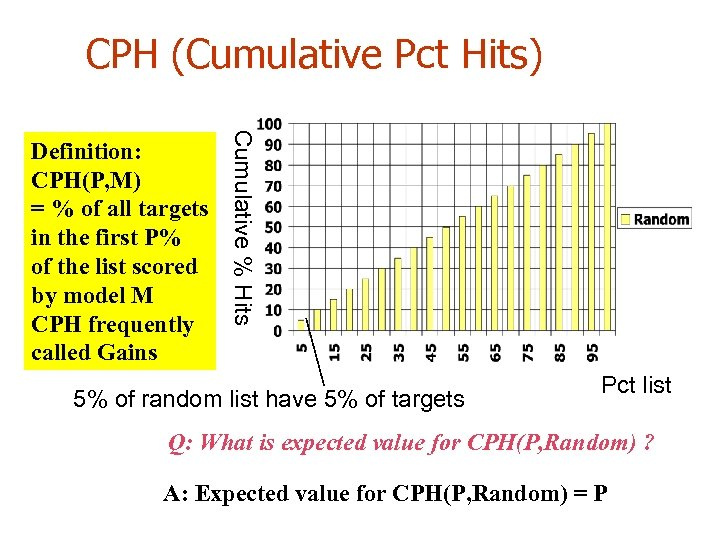CPH (Cumulative Pct Hits) Cumulative % Hits Definition: CPH(P, M) = % of all targets in the first P% of the list scored by model M CPH frequently called Gains 5% of random list have 5% of targets Pct list Q: What is expected value for CPH(P, Random) ? A: Expected value for CPH(P, Random) = P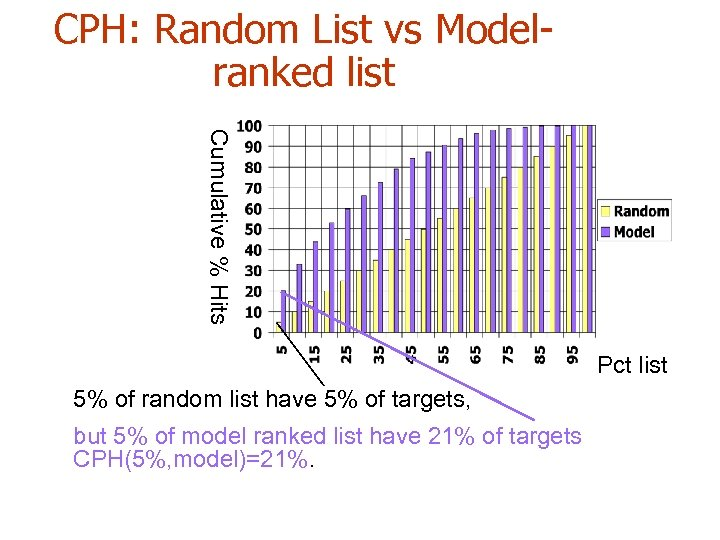CPH: Random List vs Modelranked list Cumulative % Hits Pct list 5% of random list have 5% of targets, but 5% of model ranked list have 21% of targets CPH(5%, model)=21%.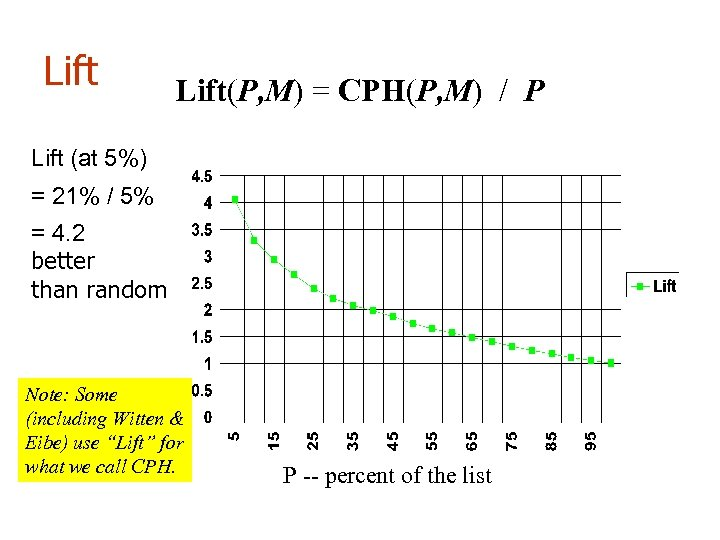Lift(P, M) = CPH(P, M) / P Lift (at 5%) = 21% / 5% = 4. 2 better than random Note: Some (including Witten & Eibe) use “Lift” for what we call CPH. P -- percent of the list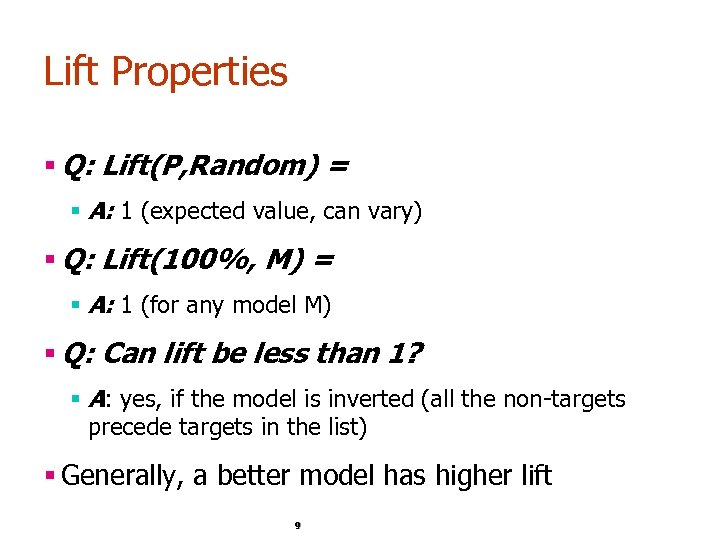Lift Properties § Q: Lift(P, Random) = § A: 1 (expected value, can vary) § Q: Lift(100%, M) = § A: 1 (for any model M) § Q: Can lift be less than 1? § A: yes, if the model is inverted (all the non-targets precede targets in the list) § Generally, a better model has higher lift 9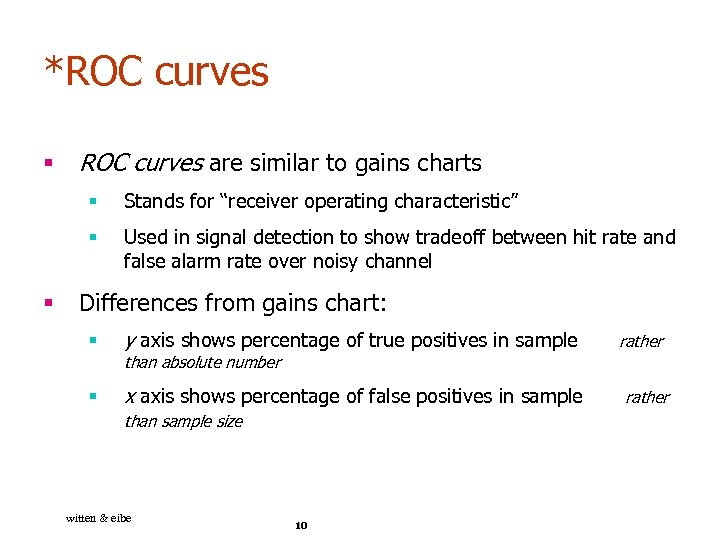*ROC curves § ROC curves are similar to gains charts § § § Stands for “receiver operating characteristic” Used in signal detection to show tradeoff between hit rate and false alarm rate over noisy channel Differences from gains chart: § y axis shows percentage of true positives in sample § x axis shows percentage of false positives in sample than absolute number than sample size witten & eibe 10 rather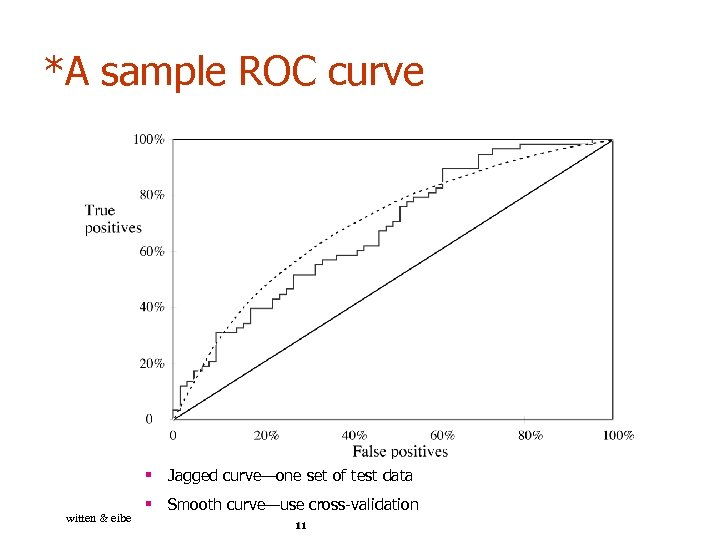*A sample ROC curve § Jagged curve—one set of test data witten & eibe § Smooth curve—use cross-validation 11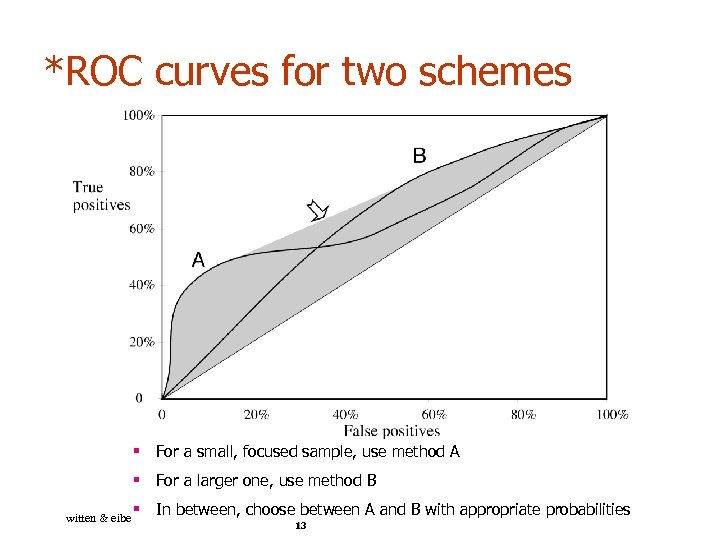*ROC curves for two schemes § For a small, focused sample, use method A § For a larger one, use method B witten & eibe § In between, choose between A and B with appropriate probabilities 13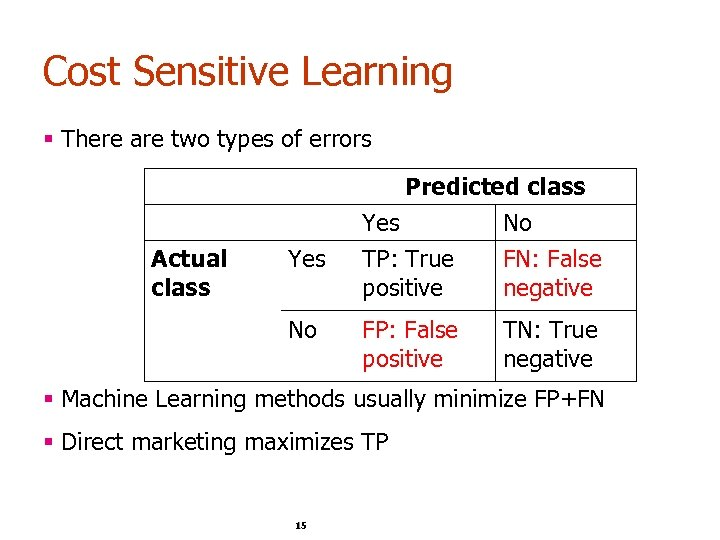Cost Sensitive Learning § There are two types of errors Actual class Yes No Predicted class Yes No TP: True FN: False positive negative FP: False positive TN: True negative § Machine Learning methods usually minimize FP+FN § Direct marketing maximizes TP 15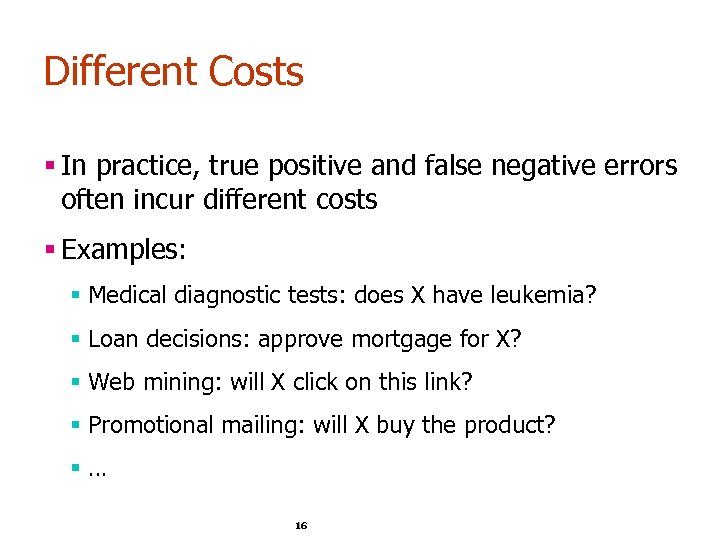Different Costs § In practice, true positive and false negative errors often incur different costs § Examples: § Medical diagnostic tests: does X have leukemia? § Loan decisions: approve mortgage for X? § Web mining: will X click on this link? § Promotional mailing: will X buy the product? §… 16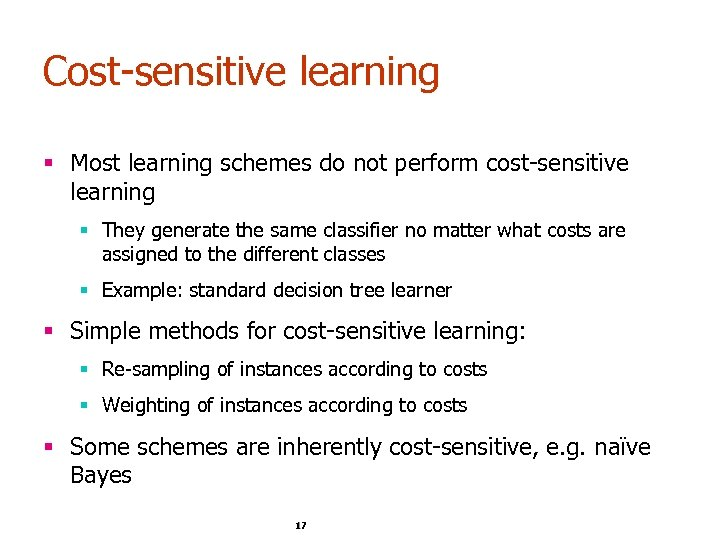Cost-sensitive learning § Most learning schemes do not perform cost-sensitive learning § They generate the same classifier no matter what costs are assigned to the different classes § Example: standard decision tree learner § Simple methods for cost-sensitive learning: § Re-sampling of instances according to costs § Weighting of instances according to costs § Some schemes are inherently cost-sensitive, e. g. naïve Bayes 17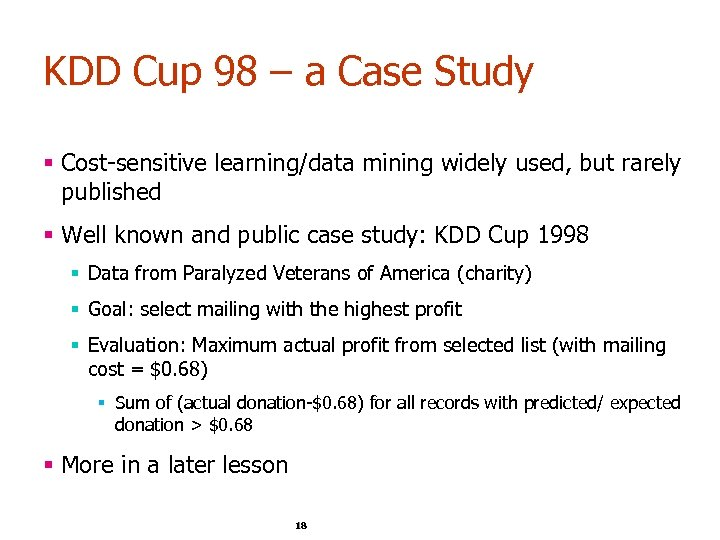KDD Cup 98 – a Case Study § Cost-sensitive learning/data mining widely used, but rarely published § Well known and public case study: KDD Cup 1998 § Data from Paralyzed Veterans of America (charity) § Goal: select mailing with the highest profit § Evaluation: Maximum actual profit from selected list (with mailing cost = \$0. 68) § Sum of (actual donation-\$0. 68) for all records with predicted/ expected donation > \$0. 68 § More in a later lesson 18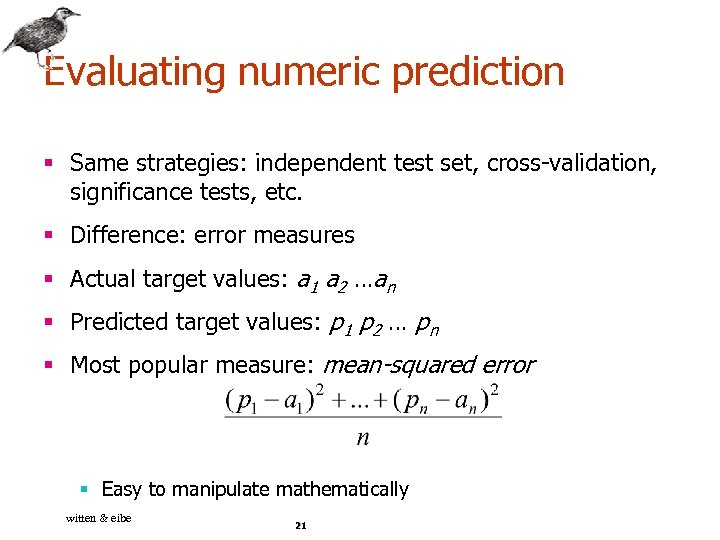Evaluating numeric prediction § Same strategies: independent test set, cross-validation, significance tests, etc. § Difference: error measures § Actual target values: a 1 a 2 …an § Predicted target values: p 1 p 2 … pn § Most popular measure: mean-squared error § Easy to manipulate mathematically witten & eibe 21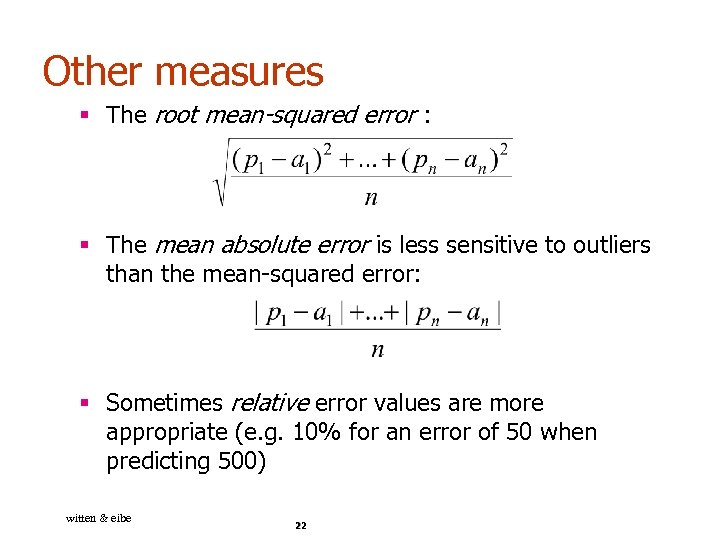Other measures § The root mean-squared error : § The mean absolute error is less sensitive to outliers than the mean-squared error: § Sometimes relative error values are more appropriate (e. g. 10% for an error of 50 when predicting 500) witten & eibe 22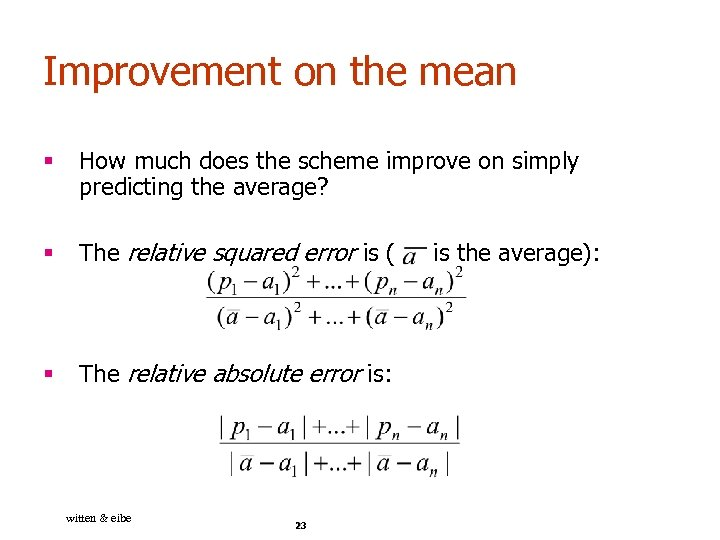Improvement on the mean § How much does the scheme improve on simply predicting the average? § The relative squared error is ( § The relative absolute error is: witten & eibe 23 is the average):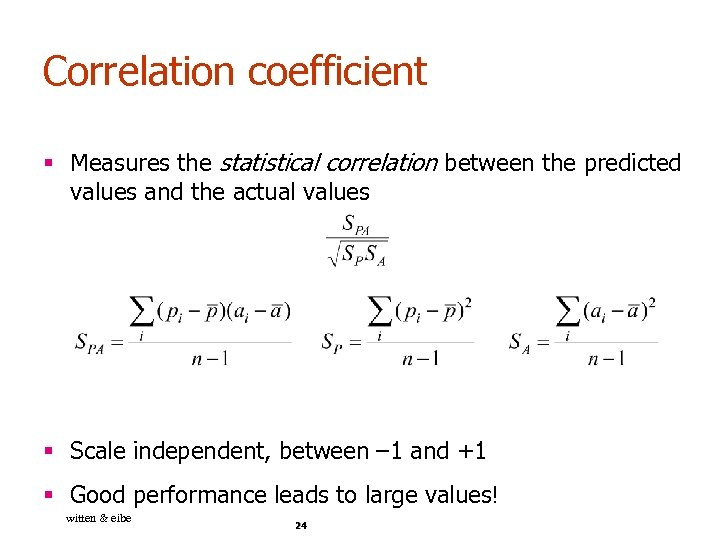Correlation coefficient § Measures the statistical correlation between the predicted values and the actual values § Scale independent, between – 1 and +1 § Good performance leads to large values! witten & eibe 24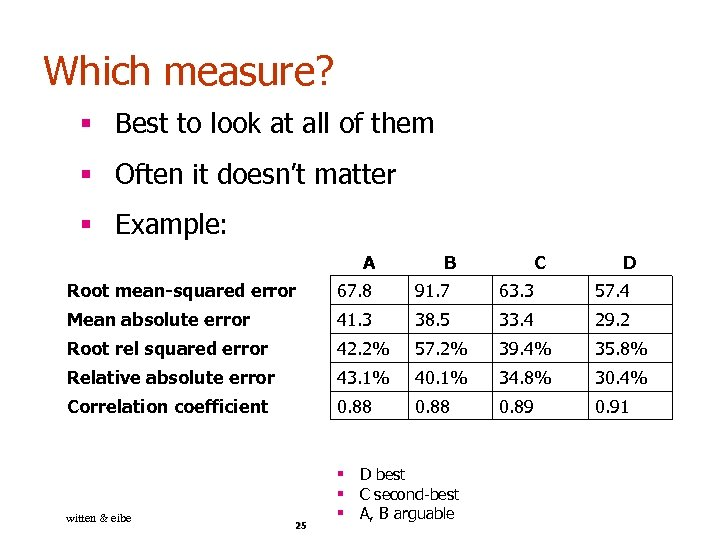Which measure? § Best to look at all of them § Often it doesn’t matter § Example: A B C D Root mean-squared error 67. 8 91. 7 63. 3 57. 4 Mean absolute error 41. 3 38. 5 33. 4 29. 2 Root rel squared error 42. 2% 57. 2% 39. 4% 35. 8% Relative absolute error 43. 1% 40. 1% 34. 8% 30. 4% Correlation coefficient 0. 88 0. 89 0. 91 witten & eibe § D best § C second-best § A, B arguable 25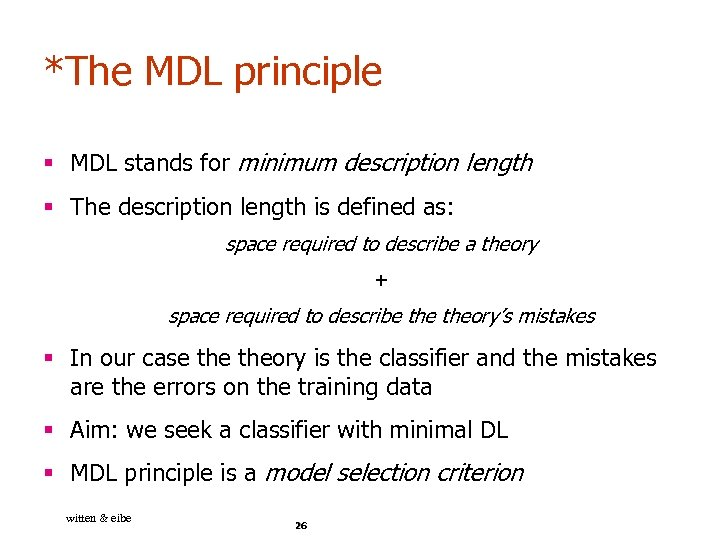*The MDL principle § MDL stands for minimum description length § The description length is defined as: space required to describe a theory + space required to describe theory’s mistakes § In our case theory is the classifier and the mistakes are the errors on the training data § Aim: we seek a classifier with minimal DL § MDL principle is a model selection criterion witten & eibe 26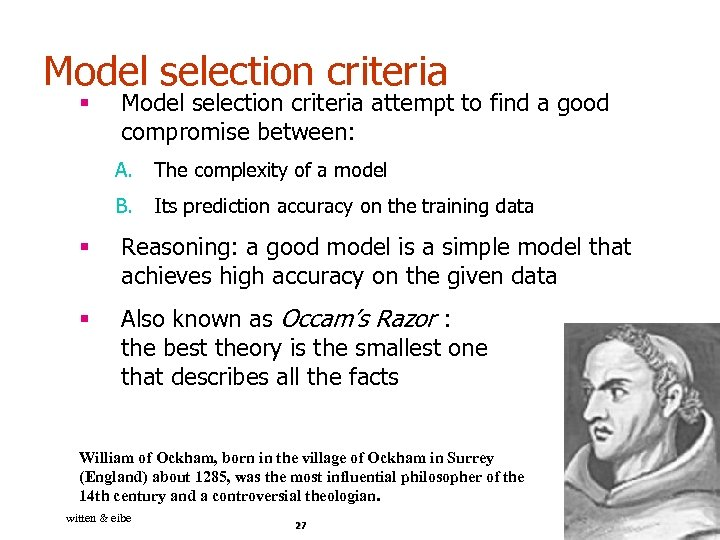Model selection criteria § Model selection criteria attempt to find a good compromise between: A. The complexity of a model B. Its prediction accuracy on the training data § Reasoning: a good model is a simple model that achieves high accuracy on the given data § Also known as Occam’s Razor : the best theory is the smallest one that describes all the facts William of Ockham, born in the village of Ockham in Surrey (England) about 1285, was the most influential philosopher of the 14 th century and a controversial theologian. witten & eibe 27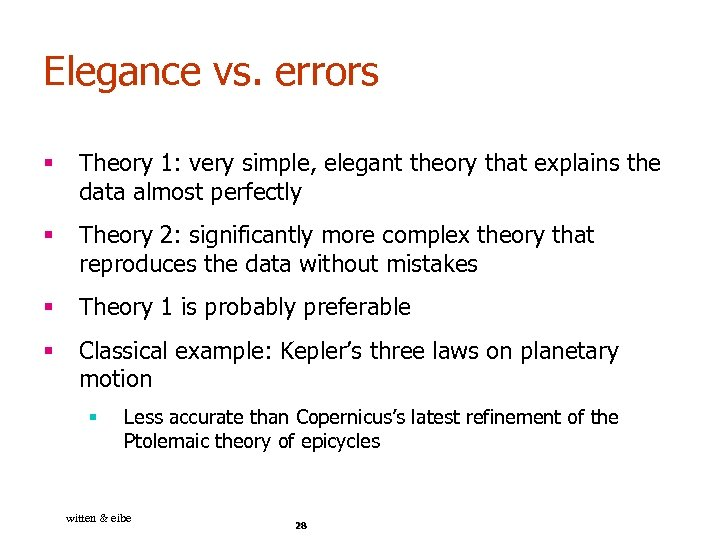Elegance vs. errors § Theory 1: very simple, elegant theory that explains the data almost perfectly § Theory 2: significantly more complex theory that reproduces the data without mistakes § Theory 1 is probably preferable § Classical example: Kepler’s three laws on planetary motion § Less accurate than Copernicus’s latest refinement of the Ptolemaic theory of epicycles witten & eibe 28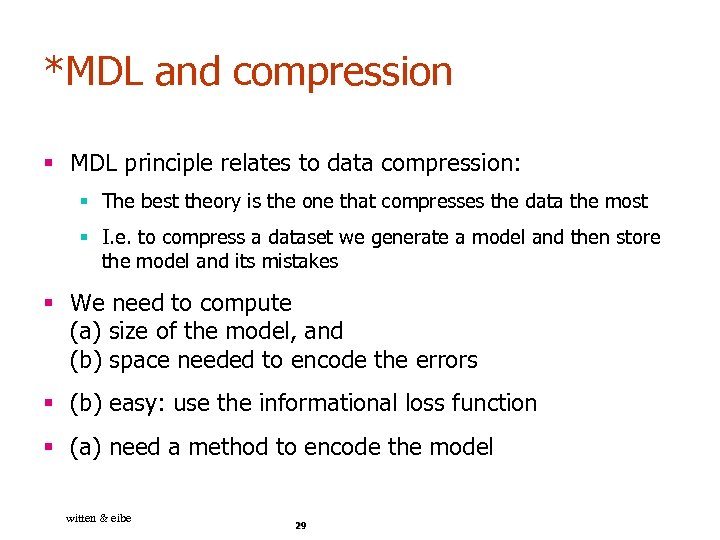*MDL and compression § MDL principle relates to data compression: § The best theory is the one that compresses the data the most § I. e. to compress a dataset we generate a model and then store the model and its mistakes § We need to compute (a) size of the model, and (b) space needed to encode the errors § (b) easy: use the informational loss function § (a) need a method to encode the model witten & eibe 29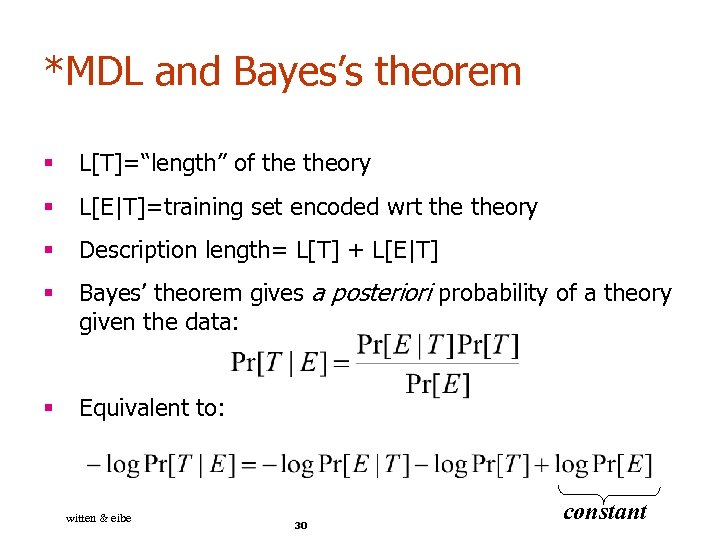*MDL and Bayes’s theorem § L[T]=“length” of theory § L[E|T]=training set encoded wrt theory § Description length= L[T] + L[E|T] § Bayes’ theorem gives a posteriori probability of a theory given the data: § Equivalent to: witten & eibe 30 constant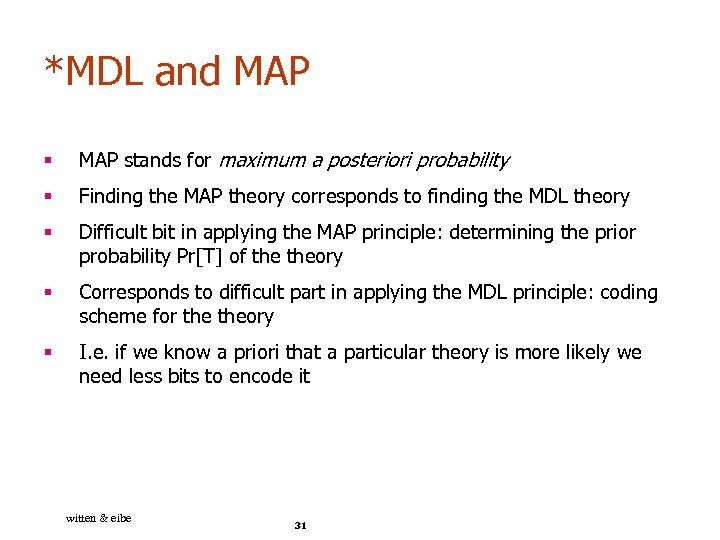*MDL and MAP § MAP stands for maximum a posteriori probability § Finding the MAP theory corresponds to finding the MDL theory § Difficult bit in applying the MAP principle: determining the prior probability Pr[T] of theory § Corresponds to difficult part in applying the MDL principle: coding scheme for theory § I. e. if we know a priori that a particular theory is more likely we need less bits to encode it witten & eibe 31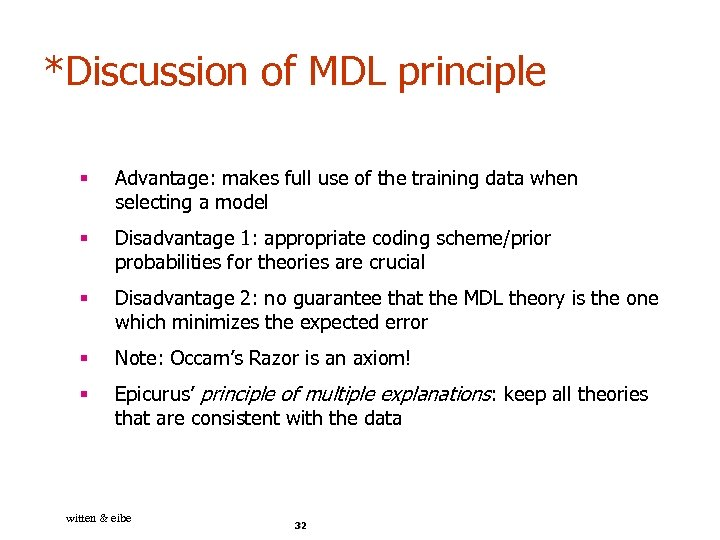*Discussion of MDL principle § Advantage: makes full use of the training data when selecting a model § Disadvantage 1: appropriate coding scheme/prior probabilities for theories are crucial § Disadvantage 2: no guarantee that the MDL theory is the one which minimizes the expected error § Note: Occam’s Razor is an axiom! § Epicurus’ principle of multiple explanations: keep all theories that are consistent with the data witten & eibe 32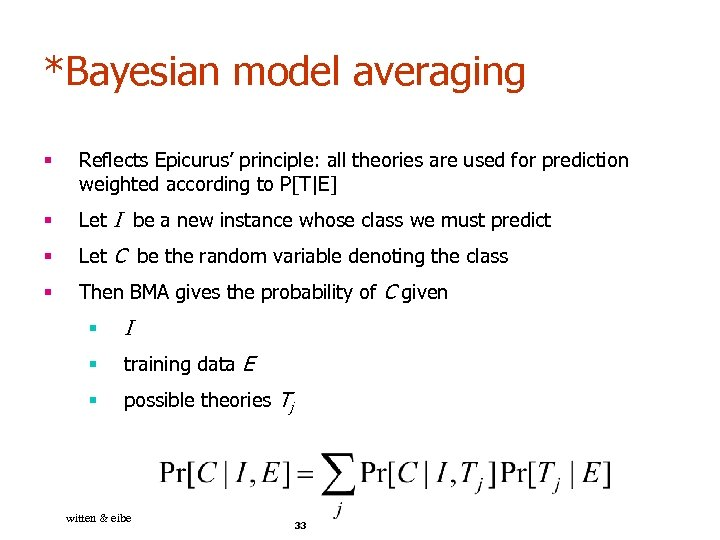*Bayesian model averaging § Reflects Epicurus’ principle: all theories are used for prediction weighted according to P[T|E] § Let I be a new instance whose class we must predict § Let C be the random variable denoting the class § Then BMA gives the probability of C given § I § training data E § possible theories Tj witten & eibe 33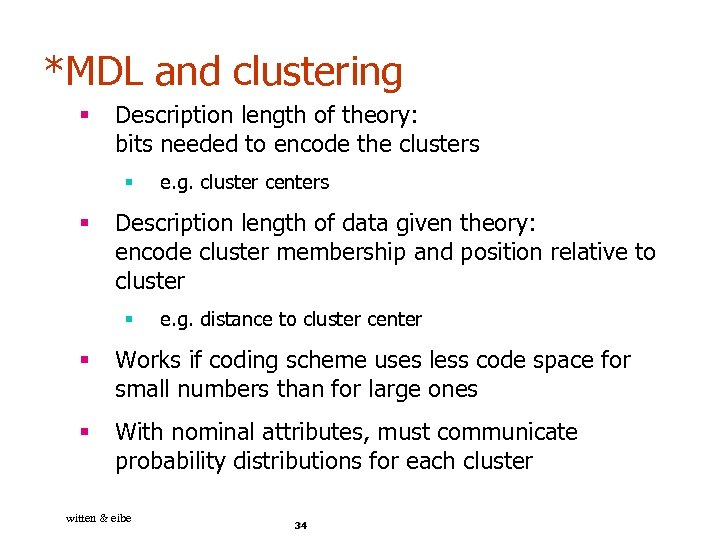*MDL and clustering § Description length of theory: bits needed to encode the clusters § § e. g. cluster centers Description length of data given theory: encode cluster membership and position relative to cluster § e. g. distance to cluster center § Works if coding scheme uses less code space for small numbers than for large ones § With nominal attributes, must communicate probability distributions for each cluster witten & eibe 34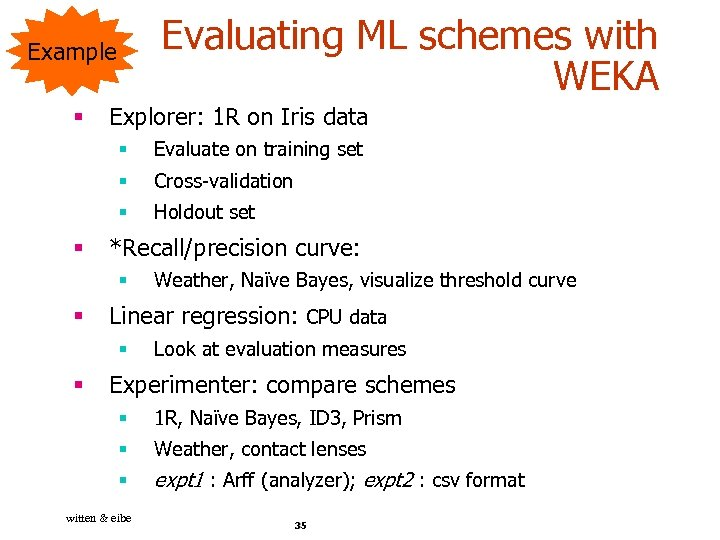Evaluating ML schemes with WEKA Example § Explorer: 1 R on Iris data § § Cross-validation § § Evaluate on training set Holdout set *Recall/precision curve: § § Linear regression: CPU data § § Weather, Naïve Bayes, visualize threshold curve Look at evaluation measures Experimenter: compare schemes § 1 R, Naïve Bayes, ID 3, Prism § Weather, contact lenses § expt 1 : Arff (analyzer); expt 2 : csv format witten & eibe 35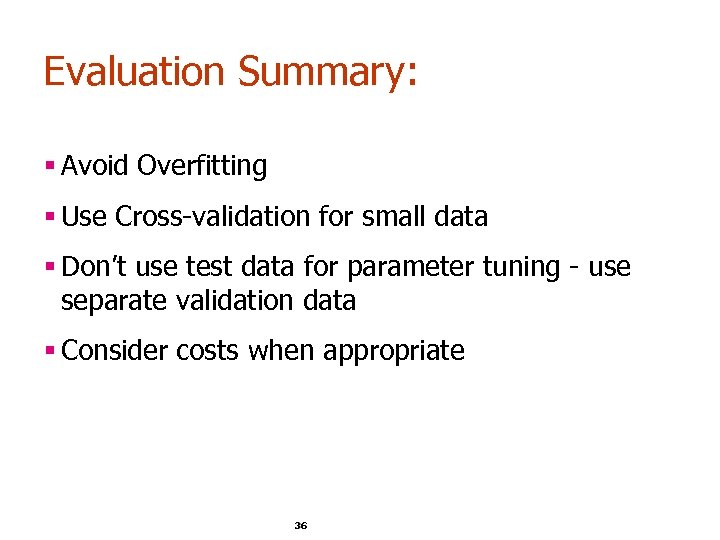Evaluation Summary: § Avoid Overfitting § Use Cross-validation for small data § Don’t use test data for parameter tuning - use separate validation data § Consider costs when appropriate 36